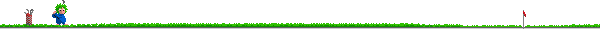GOLF BALL PHYSICS

A quick way to measure gIt is alleged that here on this planet the acceleration due to gravity is some value close to . g, = 9.8 m/s^2. This quantity has been measured by any number of ways. Let's try a rather straightforward method here.

1.Bind two meter sticks together end-to-end using a 1/2 meter stick as a splint. Tape or otherwise affix your new measuring device to a wall or table so that it stands vertically from the floor without being held by you or your partner.

2 Select a distance h1, 1m < h1 <2m, from which to drop your golf ball. Note that while h1 will not directly enter into your calculations, you should note what h1 is so you can drop the ball repeatedly from the same distance h1.

3. Drop your ball from h1. This part is tricky. The ball will hit the floor, rise to some height h2, and fall to the floor again. One of your partnership will have to measure h2, while the other measures the time between bounces. Practice this routine as many times as necessary to develop some proficiency and some consistency.

4. Once you are confident that you can measure what needs to be measured, proceed as follows. From a given starting height, h1, drop the ball at least ten times. For each trial, record the height of rise, h2, as well as the flight time, t, between bounces. From these measurements, calculate the acceleration (g) that the ball experienced between bounces. Report your data for h2, t, and g in a suitable table. Use Excel to execute the calculations.

5. Repeat step 4 for two more trials, using starting heights h1' = 1.25 h1,
and h1" = .75 h
1. Record the data as before.

6. Are there any differences among the results of the three sets of trials? Should there be?

A written report outlining your findings, prepared in the usual way, is due five class days from today.